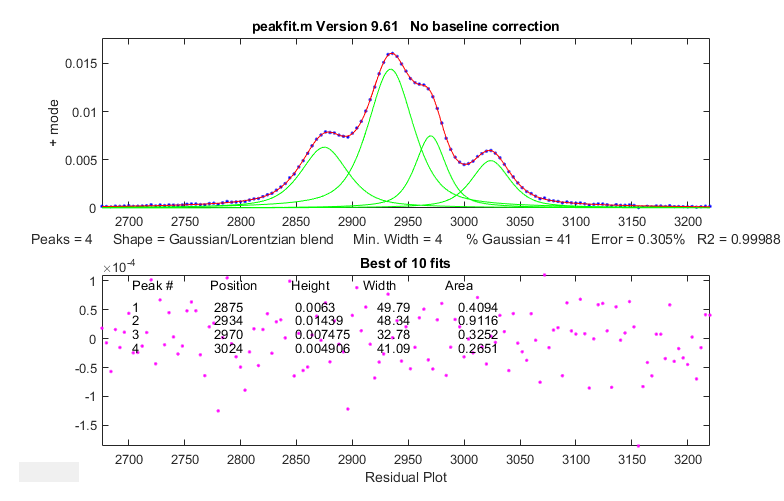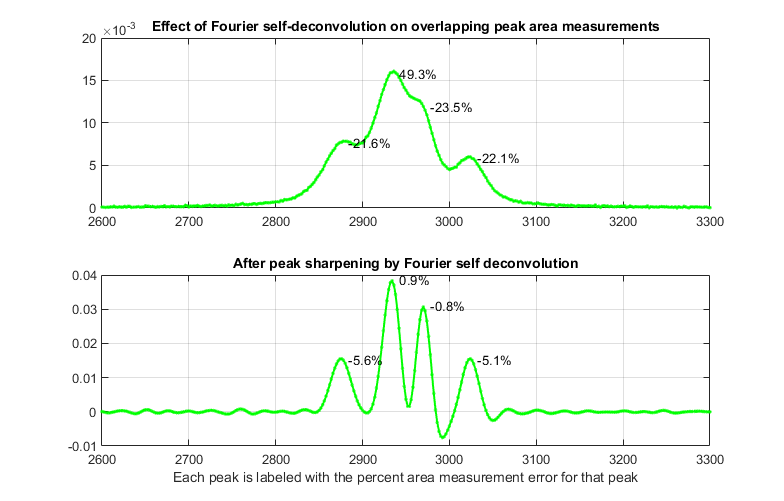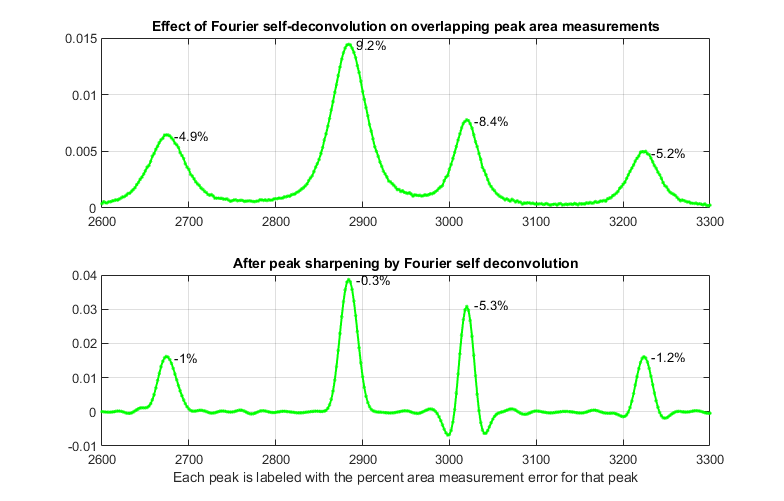[Introduction]  [Signal arithmetic]  [Signals and noise]   [Smoothing]   [Differentiation]  [Peak Sharpening]  [Harmonic analysis]   [Fourier convolution]  [Fourier deconvolution]  [Fourier filter]  [Wavelets]   [Peak area measurement]  [Linear Least Squares]  [Multicomponent Spectroscopy]  [Iterative Curve Fitting]  [Hyperlinear quantitative absorption spectrophotometry] [Appendix and Case Studies]  [Peak Finding and Measurement]  [iPeak]   [iSignal]  [Peak Fitters]   [iFilter]  [iPower]  [List of downloadable software]  [Interactive tools]

## Appendix AG. Using real-signal modeling to determine measurement accuracy

It's common to use computer-generated signals whose characteristics are known exactly to establish the accuracy of a proposed signal processing method, analogous to the use of standards in analytical chemistry. But the problem with computer-generated signals is that they are often too simple or too ideal, such as a series of peaks that are all equal in height and width, of some idealized shape such as a pure Gaussian, and with idealized added random white noise. For the measurement of the areas of partly overlapping peaks, such ideal peaks will result in overly optimistic estimates of area measurement accuracy. One way to create more realistic known signals for a particular application is to use iterative curve fitting. If it is possible to find a model that fits the experimental data very well, with very low fitting error and with random residuals, then the peak parameters from that fit are used to construct a more realistic synthetic signal that yield a much better evaluation of the measurement. Moreover, synthetic signals can be modified at will to explore how the proposed measurement method might work under other experimental conditions (e.g., if the sampling frequency were higher).To demonstrate this idea, I downloaded a spectrum from the NIST IR database that contained a set of four highly fused peaks. To determine the true peak areas as accurately as possible, I iteratively fit those peaks with four GLS peaks (41% Gaussian) of different widths, yielding a fitting error of only 0.3%, and an R2 of 0.99988, with unstructured random residuals, as shown on the left. The best-fit peak parameters and the residual noise were then used in a self-contained script to create a synthetic model signal that is essentially identical to the experimental spectrum, except that it has exactly known peak areas. Then, the script uses the simpler and faster perpendicular drop method to measure those areas, using second differentiation to locate the original peak positions, peak area measurement by perpendicular drop, which by itself is not expected to work on such overlapped peaks, and finally repeating the area measurements after sharpening the peaks by Fourier self-deconvolution, using a low-pass Fourier filter to control the noise).

As shown by the first figure below, self-deconvolution sharpening can in fact improve the peak area accuracy substantially, from an average error of 29% for the original signal to only 3.1% after deconvolution. But because the peaks have different widths, there is no single optimum deconvolution width. Tests show that the best overall results are obtained when the deconvolution function shape is the same as in the original signal and when the deconvolution function width is 1.1 times the average peak width in the signal. In the second figure, the peaks in the model signal have been spread out artificially, with no other change, just to show more clearly that this choice of deconvolution function width causes the third peak to be "over sharpened", resulting in negative lobes for that peak. (But recall that deconvolution is done in a way that conserves total peak area). A more conservative approach, using the largest deconvolution width possible without the signal ever going negative (about 0.8 times the average peak width in this case) results in only a modest improvement in area accuracy (from 27% to 12%; graphic).Sampling interval (cm-1)= 2

Change in peak separation (PeakSpread)= 0
Noise= 5e-05
GLS Shape (fraction Gaussian)= 0.41
Deconvolution Width= 23.7 points
(1.1 times the mean signal peak width)

Frequency Cutoff= 20%

\Change in peak separation ("PeakSpread") = 100. All other parameters are unchanged.

This page is part of "A Pragmatic Introduction to Signal Processing", created and maintained by Prof. Tom O'Haver , Department of Chemistry and Biochemistry, The University of Maryland at College Park. Comments, suggestions and questions should be directed to Prof. O'Haver at toh@umd.edu. Updated January, 2023.